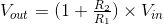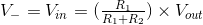# Non-Inverting Amplifier

## Working principle

The non-inverting amplifier configuration produces an output voltage, which is equal to its input voltage scaled by a voltage-divider ratio due to its resistors:### Formula derivation

Since the op-amp tries to keep its two terminals at an equal voltage, the inverting input of the op-amp (V-) is at the same potential as the non-inverting terminal (V+) and, thus, is equal to the input voltage: V- = V+ = Vin.

The output voltage can be found by using the voltage-divider network due to R1 and R2:### Experiments

• Vary the input voltage and resistance values and observe the change at the output.
• Change the input voltage to a negative value and verify that the output voltage is now negative as well.
• Make the resistor values equal. What is the output voltage now in terms of the input voltage?